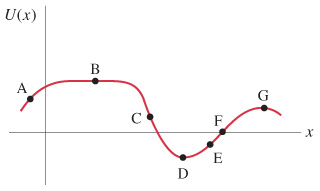# Problem: The figure shows a potential energy curve, U(x). At which point does the force have greatest magnitude?For each labeled point, state whether the force acts to the left or to the right, or is zero.Where is there equilibrium and of what type is it?

###### FREE Expert Solution
97% (258 ratings)
###### Problem Details

The figure shows a potential energy curve, U(x).At which point does the force have greatest magnitude?

For each labeled point, state whether the force acts to the left or to the right, or is zero.

Where is there equilibrium and of what type is it?